# Chemial kinetic problem.

4 de Aug de 2016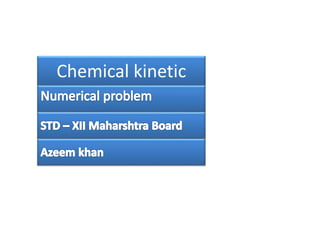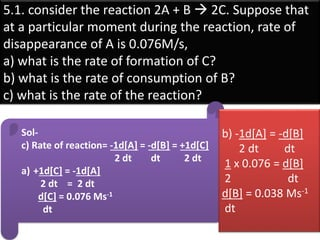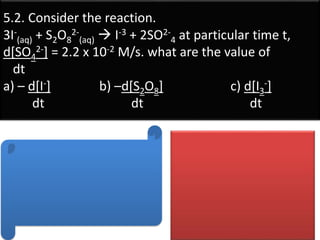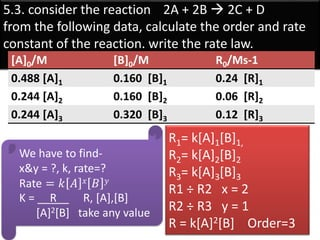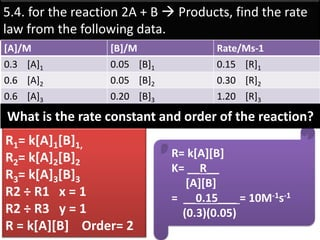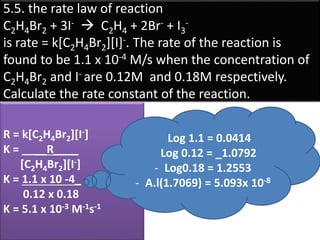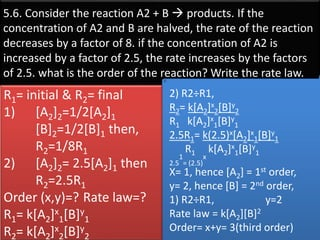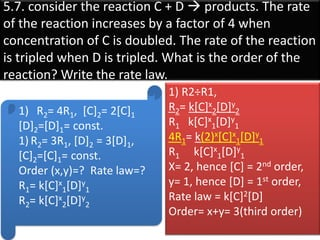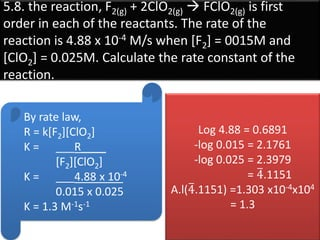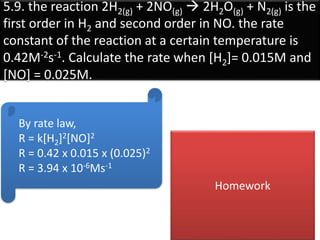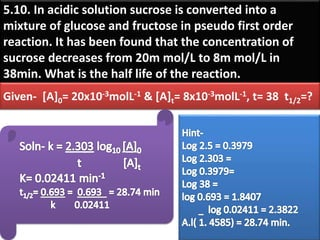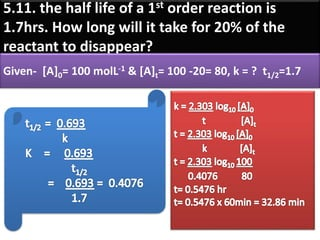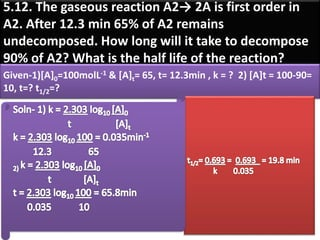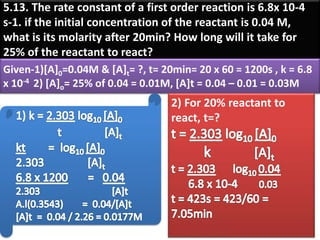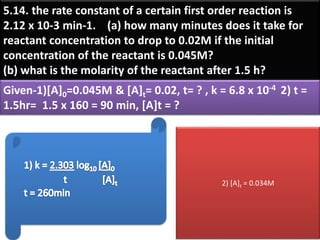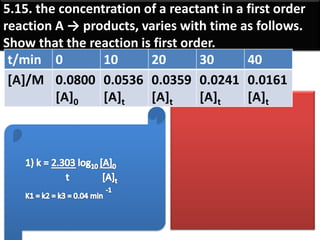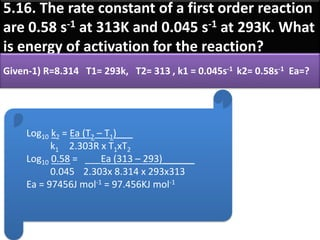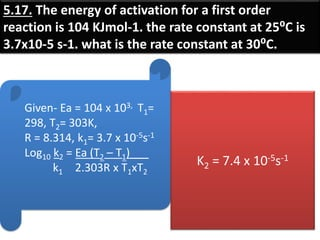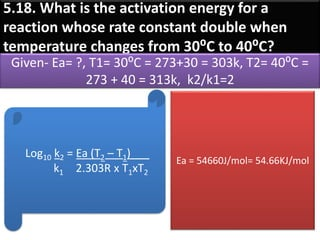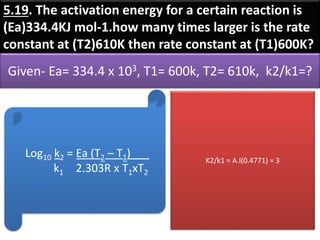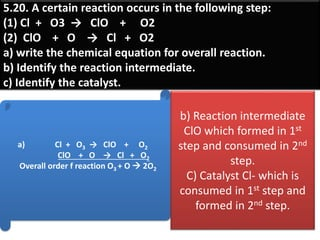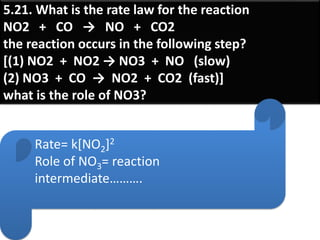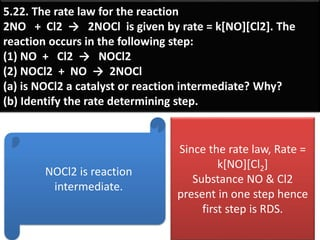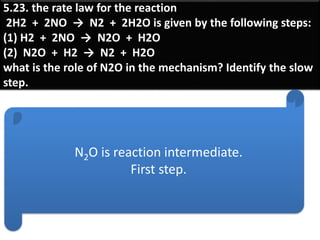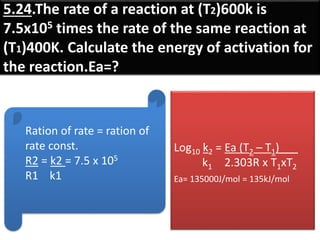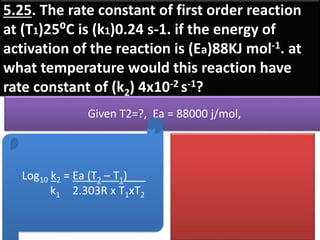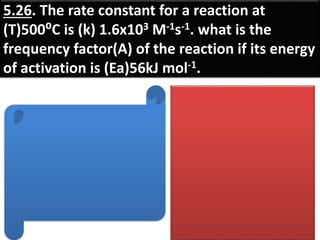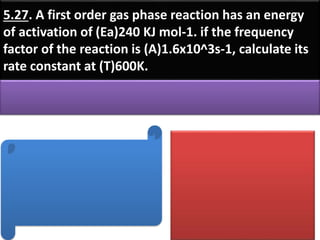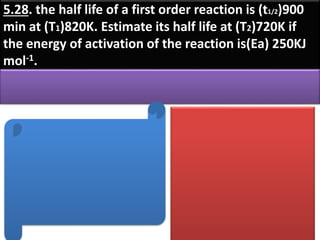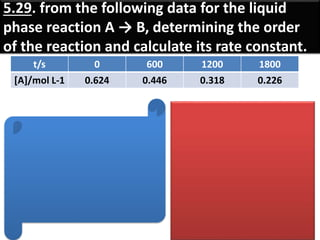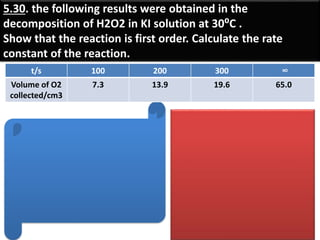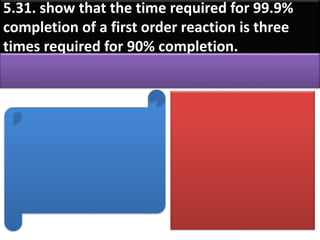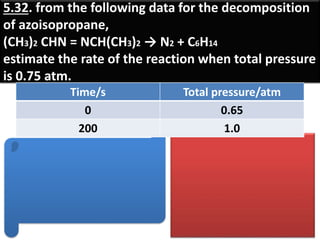1 de 33

### Chemial kinetic problem.

• 2. 5.1. consider the reaction 2A + B  2C. Suppose that at a particular moment during the reaction, rate of disappearance of A is 0.076M/s, a) what is the rate of formation of C? b) what is the rate of consumption of B? c) what is the rate of the reaction? Sol- c) Rate of reaction= -1d[A] = -d[B] = +1d[C] 2 dt dt 2 dt a) +1d[C] = -1d[A] 2 dt = 2 dt d[C] = 0.076 Ms-1 dt b) -1d[A] = -d[B] 2 dt dt 1 x 0.076 = d[B] 2 dt d[B] = 0.038 Ms-1 dt
• 3. 5.2. Consider the reaction. 3I- (aq) + S2O8 2- (aq)  I-3 + 2SO2- 4 at particular time t, d[SO4 2-] = 2.2 x 10-2 M/s. what are the value of dt a) – d[I-] b) –d[S2O8] c) d[I3 -] dt dt dt
• 4. 5.3. consider the reaction 2A + 2B  2C + D from the following data, calculate the order and rate constant of the reaction. write the rate law. We have to find- x&y = ?, k, rate=? Rate = 𝑘 𝐴 𝑥 𝐵 𝑦 K = __R__ R, [A],[B] [A]2[B] take any value R1= k[A]1[B]1, R2= k[A]2[B]2 R3= k[A]3[B]3 R1 ÷ R2 x = 2 R2 ÷ R3 y = 1 R = k[A]2[B] Order=3 [A]0/M [B]0/M R0/Ms-1 0.488 [A]1 0.160 [B]1 0.24 [R]1 0.244 [A]2 0.160 [B]2 0.06 [R]2 0.244 [A]3 0.320 [B]3 0.12 [R]3
• 5. 5.4. for the reaction 2A + B  Products, find the rate law from the following data. R= k[A][B] K= __R__ [A][B] = __0.15___ = 10M-1s-1 (0.3)(0.05) R1= k[A]1[B]1, R2= k[A]2[B]2 R3= k[A]3[B]3 R2 ÷ R1 x = 1 R2 ÷ R3 y = 1 R = k[A][B] Order= 2 [A]/M [B]/M Rate/Ms-1 0.3 [A]1 0.05 [B]1 0.15 [R]1 0.6 [A]2 0.05 [B]2 0.30 [R]2 0.6 [A]3 0.20 [B]3 1.20 [R]3 What is the rate constant and order of the reaction?
• 6. 5.5. the rate law of reaction C2H4Br2 + 3I-  C2H4 + 2Br- + I3 - is rate = k[C2H4Br2][I]-. The rate of the reaction is found to be 1.1 x 10-4 M/s when the concentration of C2H4Br2 and I- are 0.12M and 0.18M respectively. Calculate the rate constant of the reaction. R = k[C2H4Br2][I-] K = ____R____ [C2H4Br2][I-] K = 1.1 x 10 -4_ 0.12 x 0.18 K = 5.1 x 10-3 M-1s-1 Log 1.1 = 0.0414 Log 0.12 = _1.0792 - Log0.18 = 1.2553 - A.l(1.7069) = 5.093x 10-8
• 7. 5.6. Consider the reaction A2 + B  products. If the concentration of A2 and B are halved, the rate of the reaction decreases by a factor of 8. if the concentration of A2 is increased by a factor of 2.5, the rate increases by the factors of 2.5. what is the order of the reaction? Write the rate law. 2) R2÷R1, R2= k[A2]x 2[B]y 2 R1 k[A2]x 1[B]y 1 2.5R1= k(2.5)x[A2]x 1[B]y 1 R1 k[A2]x 1[B]y 1 2.5 1 = (2.5) x X= 1, hence [A2] = 1st order, y= 2, hence [B] = 2nd order, 1) R2÷R1, y=2 Rate law = k[A2][B]2 Order= x+y= 3(third order) R1= initial & R2= final 1) [A2]2=1/2[A2]1 [B]2=1/2[B]1 then, R2=1/8R1 2) [A2]2= 2.5[A2]1 then R2=2.5R1 Order (x,y)=? Rate law=? R1= k[A2]x 1[B]y 1 R2= k[A2]x 2[B]y 2
• 8. 5.7. consider the reaction C + D  products. The rate of the reaction increases by a factor of 4 when concentration of C is doubled. The rate of the reaction is tripled when D is tripled. What is the order of the reaction? Write the rate law. 1) R2= 4R1, [C]2= 2[C]1 [D]2=[D]1= const. 1) R2= 3R1, [D]2 = 3[D]1, [C]2=[C]1= const. Order (x,y)=? Rate law=? R1= k[C]x 1[D]y 1 R2= k[C]x 2[D]y 2 1) R2÷R1, R2= k[C]x 2[D]y 2 R1 k[C]x 1[D]y 1 4R1= k(2)x[C]x 1[D]y 1 R1 k[C]x 1[D]y 1 X= 2, hence [C] = 2nd order, y= 1, hence [D] = 1st order, Rate law = k[C]2[D] Order= x+y= 3(third order)
• 9. 5.8. the reaction, F2(g) + 2ClO2(g)  FClO2(g) is first order in each of the reactants. The rate of the reaction is 4.88 x 10-4 M/s when [F2] = 0015M and [ClO2] = 0.025M. Calculate the rate constant of the reaction. By rate law, R = k[F2][ClO2] K = ___R____ [F2][ClO2] K = ___4.88 x 10-4 0.015 x 0.025 K = 1.3 M-1s-1 Log 4.88 = 0.6891 -log 0.015 = 2.1761 -log 0.025 = 2.3979 = 4.1151 A.l(4.1151) =1.303 x10-4x104 = 1.3
• 10. 5.9. the reaction 2H2(g) + 2NO(g)  2H2O(g) + N2(g) is the first order in H2 and second order in NO. the rate constant of the reaction at a certain temperature is 0.42M-2s-1. Calculate the rate when [H2]= 0.015M and [NO] = 0.025M. By rate law, R = k[H2]2[NO]2 R = 0.42 x 0.015 x (0.025)2 R = 3.94 x 10-6Ms-1 Homework
• 11. 5.10. In acidic solution sucrose is converted into a mixture of glucose and fructose in pseudo first order reaction. It has been found that the concentration of sucrose decreases from 20m mol/L to 8m mol/L in 38min. What is the half life of the reaction. Given- [A]0= 20x10-3molL-1 & [A]t= 8x10-3molL-1, t= 38 t1/2=?
• 12. 5.11. the half life of a 1st order reaction is 1.7hrs. How long will it take for 20% of the reactant to disappear? Given- [A]0= 100 molL-1 & [A]t= 100 -20= 80, k = ? t1/2=1.7
• 13. 5.12. The gaseous reaction A2→ 2A is first order in A2. After 12.3 min 65% of A2 remains undecomposed. How long will it take to decompose 90% of A2? What is the half life of the reaction? Given-1)[A]0=100molL-1 & [A]t= 65, t= 12.3min , k = ? 2) [A]t = 100-90= 10, t=? t1/2=?
• 14. 5.13. The rate constant of a first order reaction is 6.8x 10-4 s-1. if the initial concentration of the reactant is 0.04 M, what is its molarity after 20min? How long will it take for 25% of the reactant to react? Given-1)[A]0=0.04M & [A]t= ?, t= 20min= 20 x 60 = 1200s , k = 6.8 x 10-4 2) [A]o= 25% of 0.04 = 0.01M, [A]t = 0.04 – 0.01 = 0.03M 2) For 20% reactant to react, t=?
• 15. 5.14. the rate constant of a certain first order reaction is 2.12 x 10-3 min-1. (a) how many minutes does it take for reactant concentration to drop to 0.02M if the initial concentration of the reactant is 0.045M? (b) what is the molarity of the reactant after 1.5 h? Given-1)[A]0=0.045M & [A]t= 0.02, t= ? , k = 6.8 x 10-4 2) t = 1.5hr= 1.5 x 160 = 90 min, [A]t = ? 2) [A]t = 0.034M
• 16. 5.15. the concentration of a reactant in a first order reaction A → products, varies with time as follows. Show that the reaction is first order. t/min 0 10 20 30 40 [A]/M 0.0800 [A]0 0.0536 [A]t 0.0359 [A]t 0.0241 [A]t 0.0161 [A]t
• 17. 5.16. The rate constant of a first order reaction are 0.58 s-1 at 313K and 0.045 s-1 at 293K. What is energy of activation for the reaction? Given-1) R=8.314 T1= 293k, T2= 313 , k1 = 0.045s-1 k2= 0.58s-1 Ea=? Log10 k2 = Ea (T2 – T1)___ k1 2.303R x T1xT2 Log10 0.58 = ___Ea (313 – 293)______ 0.045 2.303x 8.314 x 293x313 Ea = 97456J mol-1 = 97.456KJ mol-1
• 18. 5.17. The energy of activation for a first order reaction is 104 KJmol-1. the rate constant at 25⁰C is 3.7x10-5 s-1. what is the rate constant at 30⁰C. Given- Ea = 104 x 103, T1= 298, T2= 303K, R = 8.314, k1= 3.7 x 10-5s-1 Log10 k2 = Ea (T2 – T1)___ k1 2.303R x T1xT2 K2 = 7.4 x 10-5s-1
• 19. 5.18. What is the activation energy for a reaction whose rate constant double when temperature changes from 30⁰C to 40⁰C? Given- Ea= ?, T1= 30⁰C = 273+30 = 303k, T2= 40⁰C = 273 + 40 = 313k, k2/k1=2 Log10 k2 = Ea (T2 – T1)___ k1 2.303R x T1xT2 Ea = 54660J/mol= 54.66KJ/mol
• 20. 5.19. The activation energy for a certain reaction is (Ea)334.4KJ mol-1.how many times larger is the rate constant at (T2)610K then rate constant at (T1)600K? Given- Ea= 334.4 x 103, T1= 600k, T2= 610k, k2/k1=? Log10 k2 = Ea (T2 – T1)___ k1 2.303R x T1xT2 K2/k1 = A.l(0.4771) = 3
• 21. 5.20. A certain reaction occurs in the following step: (1) Cl + O3 → ClO + O2 (2) ClO + O → Cl + O2 a) write the chemical equation for overall reaction. b) Identify the reaction intermediate. c) Identify the catalyst. a) Cl + O3 → ClO + O2 ClO + O → Cl + O2 Overall order f reaction O3 + O  2O2 b) Reaction intermediate ClO which formed in 1st step and consumed in 2nd step. C) Catalyst Cl- which is consumed in 1st step and formed in 2nd step.
• 22. 5.21. What is the rate law for the reaction NO2 + CO → NO + CO2 the reaction occurs in the following step? [(1) NO2 + NO2 → NO3 + NO (slow) (2) NO3 + CO → NO2 + CO2 (fast)] what is the role of NO3? Rate= k[NO2]2 Role of NO3= reaction intermediate……….
• 23. 5.22. The rate law for the reaction 2NO + Cl2 → 2NOCl is given by rate = k[NO][Cl2]. The reaction occurs in the following step: (1) NO + Cl2 → NOCl2 (2) NOCl2 + NO → 2NOCl (a) is NOCl2 a catalyst or reaction intermediate? Why? (b) Identify the rate determining step. NOCl2 is reaction intermediate. Since the rate law, Rate = k[NO][Cl2] Substance NO & Cl2 present in one step hence first step is RDS.
• 24. 5.23. the rate law for the reaction 2H2 + 2NO → N2 + 2H2O is given by the following steps: (1) H2 + 2NO → N2O + H2O (2) N2O + H2 → N2 + H2O what is the role of N2O in the mechanism? Identify the slow step. N2O is reaction intermediate. First step.
• 25. 5.24.The rate of a reaction at (T2)600k is 7.5x105 times the rate of the same reaction at (T1)400K. Calculate the energy of activation for the reaction.Ea=? Ration of rate = ration of rate const. R2 = k2 = 7.5 x 105 R1 k1 Log10 k2 = Ea (T2 – T1)___ k1 2.303R x T1xT2 Ea= 135000J/mol = 135kJ/mol
• 26. 5.25. The rate constant of first order reaction at (T1)25⁰C is (k1)0.24 s-1. if the energy of activation of the reaction is (Ea)88KJ mol-1. at what temperature would this reaction have rate constant of (k2) 4x10-2 s-1? Given T2=?, Ea = 88000 j/mol, Log10 k2 = Ea (T2 – T1)___ k1 2.303R x T1xT2
• 27. 5.26. The rate constant for a reaction at (T)500⁰C is (k) 1.6x103 M-1s-1. what is the frequency factor(A) of the reaction if its energy of activation is (Ea)56kJ mol-1.
• 28. 5.27. A first order gas phase reaction has an energy of activation of (Ea)240 KJ mol-1. if the frequency factor of the reaction is (A)1.6x10^3s-1, calculate its rate constant at (T)600K.
• 29. 5.28. the half life of a first order reaction is (t1/2)900 min at (T1)820K. Estimate its half life at (T2)720K if the energy of activation of the reaction is(Ea) 250KJ mol-1.
• 30. 5.29. from the following data for the liquid phase reaction A → B, determining the order of the reaction and calculate its rate constant. t/s 0 600 1200 1800 [A]/mol L-1 0.624 0.446 0.318 0.226
• 31. 5.30. the following results were obtained in the decomposition of H2O2 in KI solution at 30⁰C . Show that the reaction is first order. Calculate the rate constant of the reaction. t/s 100 200 300 ∞ Volume of O2 collected/cm3 7.3 13.9 19.6 65.0
• 32. 5.31. show that the time required for 99.9% completion of a first order reaction is three times required for 90% completion.
• 33. 5.32. from the following data for the decomposition of azoisopropane, (CH3)2 CHN = NCH(CH3)2 → N2 + C6H14 estimate the rate of the reaction when total pressure is 0.75 atm. Time/s Total pressure/atm 0 0.65 200 1.0